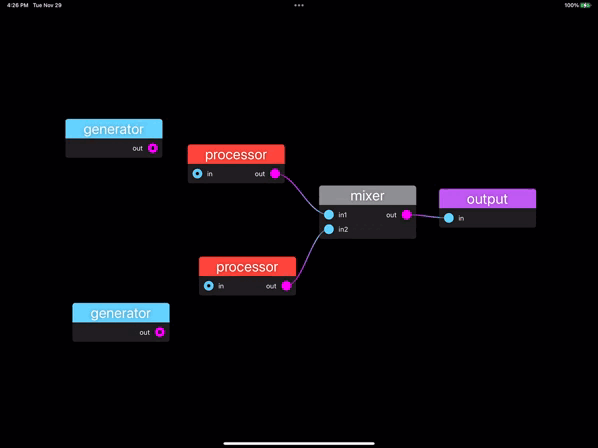# FlowGeneric node graph editor. Generate a `Patch` from your own data model. Update your data model when the `Patch` changes.```func simplePatch() -> Patch {
let generator = Node(name: "generator", outputs: ["out"])
let processor = Node(name: "processor", inputs: ["in"], outputs: ["out"])
let mixer = Node(name: "mixer", inputs: ["in1", "in2"], outputs: ["out"])
let output = Node(name: "output", inputs: ["in"])

let nodes = [generator, processor, generator, processor, mixer, output]

let wires = Set([Wire(from: OutputID(0, 0), to: InputID(1, 0)),
Wire(from: OutputID(1, 0), to: InputID(4, 0)),
Wire(from: OutputID(2, 0), to: InputID(3, 0)),
Wire(from: OutputID(3, 0), to: InputID(4, 1)),
Wire(from: OutputID(4, 0), to: InputID(5, 0))])

var patch = Patch(nodes: nodes, wires: wires)
patch.recursiveLayout(nodeIndex: 5, at: CGPoint(x: 800, y: 50))
return patch
}

struct ContentView: View {
@State var patch = simplePatch()
@State var selection = Set<NodeIndex>()

var body: some View {
NodeEditor(patch: \$patch, selection: \$selection)
.onNodeMoved { index, location in
print("Node at index \(index) moved to \(location)")
}
}
.onWireRemoved { wire in
print("Removed wire: \(wire)")
}
}
}```

## Documentation

The API Reference can be found on the AudioKit Website. Package contains a demo project and a playground to help you get started quickly.

View Github